Sorry, you do not have permission to ask a question, You must login to ask question. Become VIP Member

Do you need to remove the ads? Become VIP Member

Types of Soil tests for building construction works depend on properties of soil. Design of the foundation is based on soil test report of construction site.

Soil tests for construction of buildings or any structure is the first step in construction planning to understand the suitability of soil for proposed construction work.

Soil which is responsible for allowing the stresses coming from the structure should be well tested to give excellent performance. If soil shouldn’t tested correctly then the whole building or structure is damaged or collapsed or leaned like leaning tower of Pisa. So, soil inspection or testing is the first step to proceed any construction.## Types of Soil Tests for Building Construction

Various tests on soil are conducted to decide the quality of soil for building construction. Some tests are conducted in laboratory and some are in the field. Here we will discuss about the importance of various soil tests for building construction. The tests on soil are as follows.

1. Moisture content test
2. Atterberg limits tests
3. Specific gravity of soil
4. Dry density of soil
5. Compaction test (Proctor’s test)

### Moisture Content Test on Soil

Moisture content or water content in soil is an important parameter for building construction. It is determined by several methods and they are

• Oven drying method
• Calcium carbide method
• Torsion balance method
• Pycnometer method
• Sand bath method
• Alcohol method

Of all the above oven drying method is most common and accurate method. In this method the soil sample is taken and weighed and put it in oven and dried at 110o + 5oC. After 24 hours soil is taken out and weighed. The difference between the two weights is noted as weight of water or moisture content in the soil.### Specific Gravity Test on Soil

Specific gravity of soil is the ratio of the unit weight of soil solids to that of the water. It is determined by many methods and they are.

• Density bottle method
• Pycnometer method
• Gas jar method
• Shrinkage limit method

Density bottle method and Pycnometer method are simple and common methods. In Pycnometer method, Pycnometer is weighed in 4 different cases that is empty weight (M1), empty + dry soil (M2), empty + water + dry soil (M3) and Pycnometer filled with water (M4) at room temperature. From these 4 masses specific gravity is determined by below formula.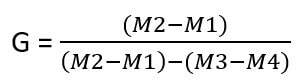### Dry Density Test on Soil

The weight of soil particles in a given volume of sample is termed as dry density of soil. Dry density of soil depends upon void ratio and specific gravity of soil. Based on values of dry density soil is classified into dense, medium dense and loose categories.

Dry density of soil is calculated by core cutter method, sand replacement method and water-displacement method.

#### Core Cutter Method for Soil Dry Density Testing

In this methods a cylindrical core cutter of standard dimensions is used to cut the soil in the ground and lift the cutter up with soil sample. The taken out sample is weighed and noted. Finally water content for that sample is determined and dry density is calculated from the below relation.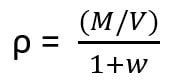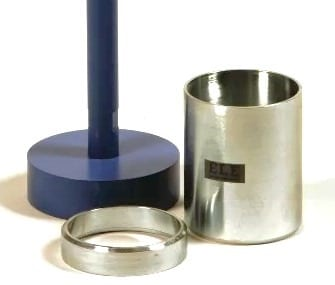#### Sand Replacement Method for Soil Dry Density Testing

In this method also, a hole is created in the ground by excavating soil whose dry density is to be find. The hole is filled with uniform sand of known dry density. So by dividing the mass of sand poured into the hole with dry density of sand gives the volume of hole. So we can calculate the soil dry density from above formula.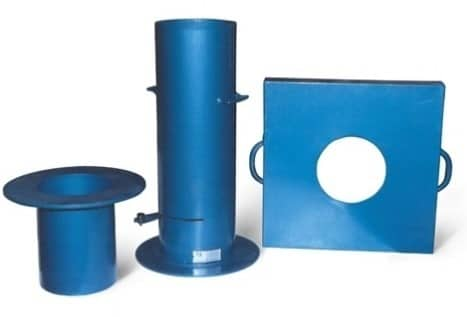### Atterberg Limits Test on Soil

To measure the critical water content of a fine grained soil, Atterberg provided 3 limits which exhibits the properties of fine grained soil at different conditions. The limits are liquid limit, plastic limit and shrinkage limit. These limits are calculated by individual tests as follows.

#### Liquid Limit Test on Soil

In this test, Casagrande’s liquid limit device is used which consist a cup with moving up and down mechanism. The cup is filled with soil sample and groove is created in the middle of cup with proper tool. When the cup is moved up and down with the help of handle the groove becomes closed at some point.

Note down the number of blows required to close the groove. After that water content of soil is determined. Repeat this procedure 3 times and draw a graph between log N and water content of soil. Water content corresponding to N=25 is the liquid limit of soil.#### Plastic Limit Test on Soil

Take the soil sample and add some water to make it plastic enough to shape into small ball. Leave it for some time and after that put that ball in the glass plate and rolled it into threads of 3mm diameter.

If the threads do not break when we roll it to below 3mm diameter, then water content is more than the plastic limit. In that case reduce water content and repeat the same procedure until crumbling occurs at 3mm diameter. Finally find out the water content of resultant soil which value is nothing but plastic limit.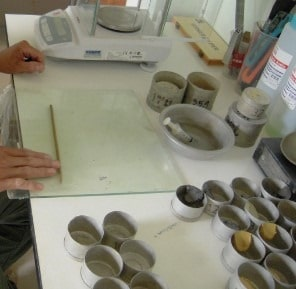#### Shrinkage Limit Test on Soil

In case of shrinkage limit, the water content in the soil is just sufficient to fill the voids of soil. That is degree of saturation is of 100%. So, there is no change in volume of soil if we reduce the shrinkage limit. It is determined by the below formula for the given soil sample.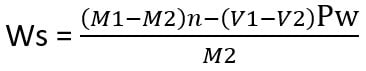Where M1 = initial mass

V1= initial volume

M2= dry mass

V2= volume after drying

Pw = density of water.

### Proctor’s Compaction Test on Soil

Proctor’s test is conducted to determine compaction characteristics of soil. Compaction of soil is nothing but reducing air voids in the soil by densification. The degree of Compaction is measured in terms of dry density of soil.In Proctor’s Compaction Test, given soil sample sieved through 20mm and 4.75 mm sieves. Percentage passing 4.75mm and percentage retained on 4.75mm are mixed with certain proportions.

Add water to it and leave it in air tight container for 20hrs. Mix the soil and divide it into 6 – 8 parts. Position the mold and pour one part of soil into the mold as 3layers with 25 blows of ramming for each layer.

Remove the base plate and Weight the soil along with mold. Remove the soil from mold and take the small portion of soil sample at different layers and conduct water content test. from the values find out the dry density of soil and water content and draw a graph between them and note down the maximum dry density and optimum water content of the compacted soil sample at highest point on the curve.

## FAQs

What are test conducted in soil before construction?

The important test conducted on soil before building construction are:
1. Moisture content test
2. Atterberg limits tests
3. Specific gravity of soil
4. Dry density of soil
5. Compaction test (Proctor’s test)

What is dry density of soil?

The weight of soil particles in a given volume of sample is termed as dry density of soil. Dry density of soil depends upon void ratio and specific gravity of soil. Based on values of dry density soil is classified into dense, medium dense and loose categories.
Dry density of soil is calculated by core cutter method, sand replacement method and water-displacement method.

How to determine moisture content of soil?

Moisture content or water content in soil is an important parameter for building construction. It is determined by several methods and they are:
1. Oven drying method
2. Calcium carbide method
3. Torsion balance method
4. Pycnometer method
5. Sand bath method
7. Alcohol method
Of all the above oven drying method is most common and accurate method. In this method the soil sample is taken and weighed and put it in oven and dried at 110o + 5oC. After 24 hours soil is taken out and weighed. The difference between the two weights is noted as weight of water or moisture content in the soil.

Types of Soil Tests for Road Construction

Soil Tests Required for Deep Foundations

Soil Tests Required for Shallow and Raft Foundations